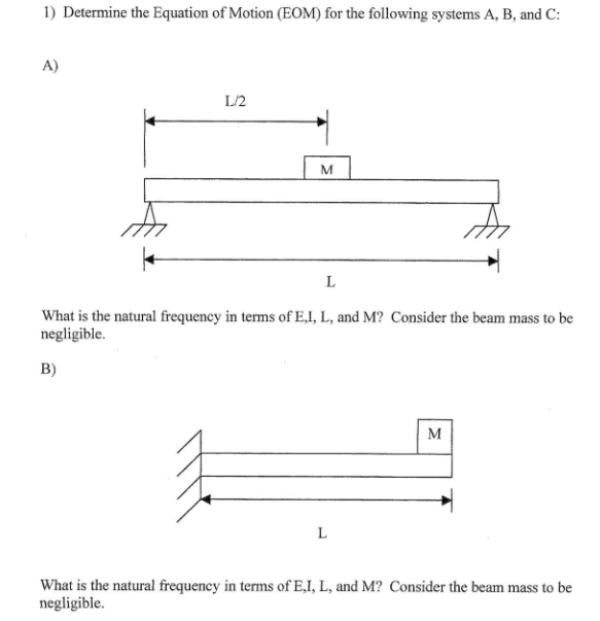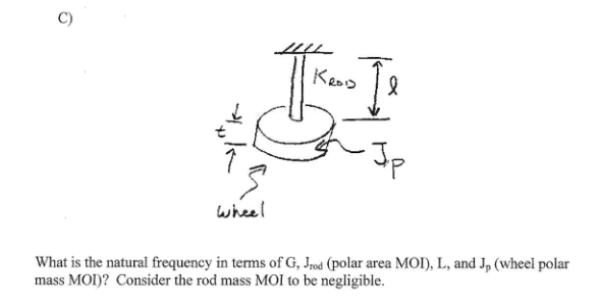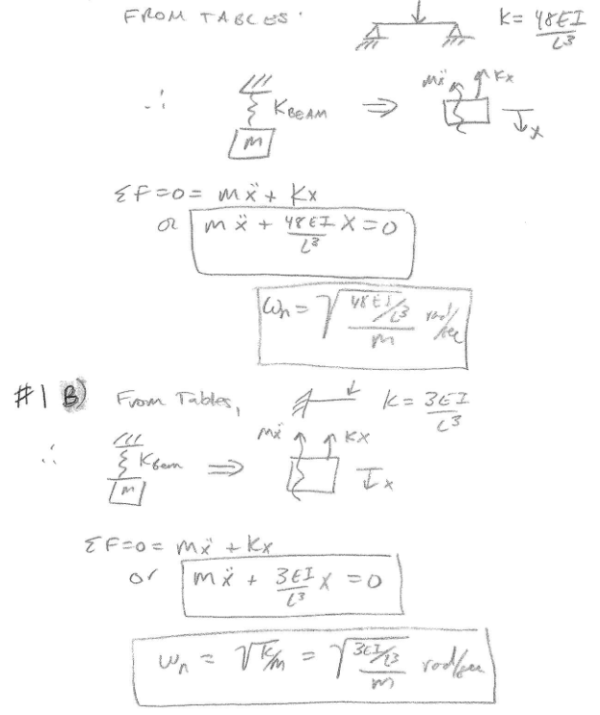Determine the equation of motion (EOM) for the following systems A, B, and C. A) What is the natural frequency in terms of E, I, L, and M? consider the beam mass to be negligible. B) What is the natural frequency in terms of E, I, L, and M? Consider the beam mass to be negligible. C) What is the natural frequency in terms of G, J (polar area MOI), L, and Jp (wheel polar mass MOI)? Consider the rod mass MOI to be negligible.Determine the equation of motion (EOM) for the following systems A, B, and C. A) What is the natural frequency in terms of E, I, L, and M? consider the beam mass to be negligible. B) What is the natural frequency in terms of E, I, L, and M? Consider the beam mass to be negligible. C) What is the natural frequency in terms of G, J (polar area MOI), L, and Jp (wheel polar mass MOI)? Consider the rod mass MOI to be negligible.

Vibrations Page 1 vibrations vibrations vibrations vibrations vibrations vibrations vibrations Vibrations Page 2